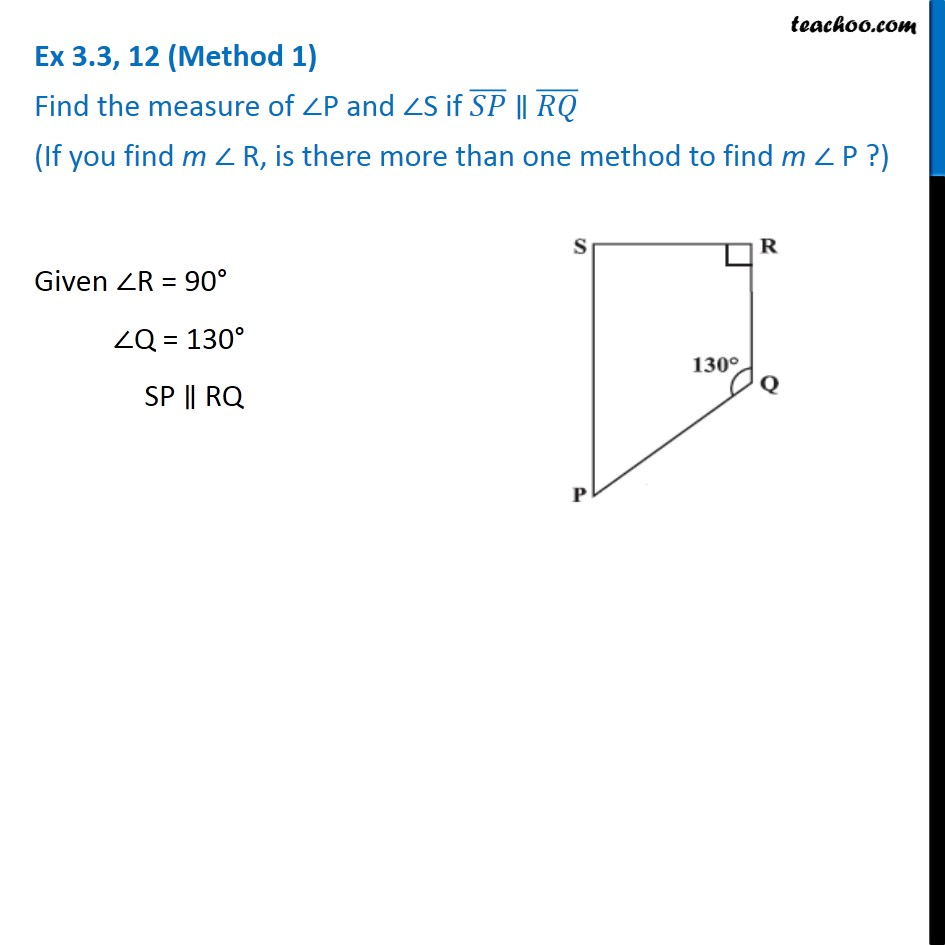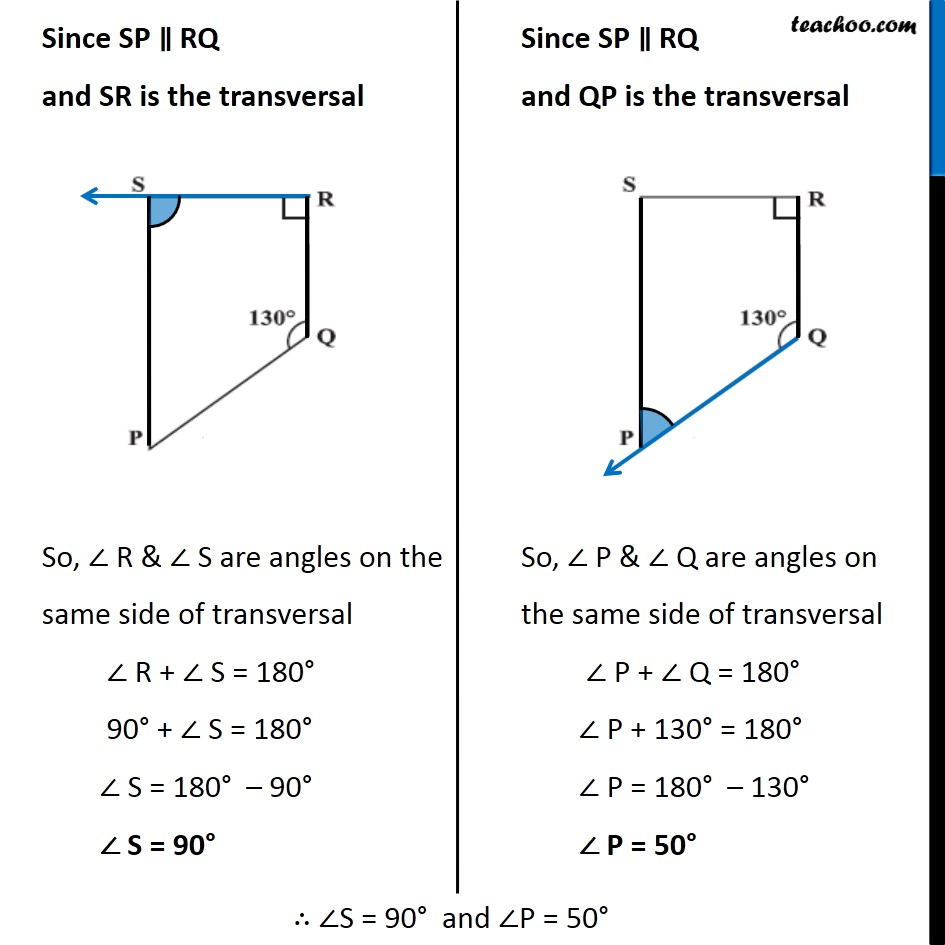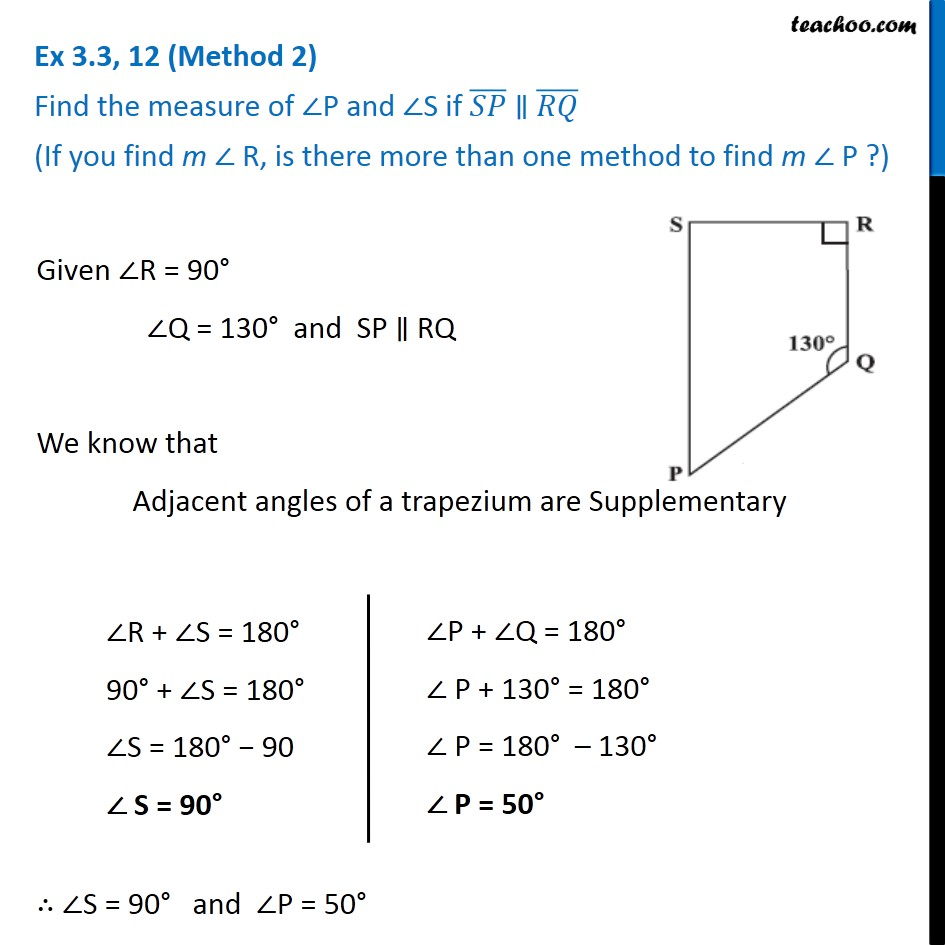Ex 3.3

Chapter 3 Class 8 Understanding Quadrilaterals
Serial order wiseLearn in your speed, with individual attention - Teachoo Maths 1-on-1 Class

### Transcript

Ex 3.3, 12 (Method 1) Find the measure of ∠P and ∠S if (𝑆𝑃) ̅ ∥ (𝑅𝑄) ̅ (If you find m ∠ R, is there more than one method to find m ∠ P ?) Given ∠R = 90° ∠Q = 130° SP ∥ RQ Since SP ∥ RQ and SR is the transversal So, ∠ R & ∠ S are angles on the same side of transversal ∠ R + ∠ S = 180° 90° + ∠ S = 180° ∠ S = 180° – 90° ∠ S = 90° Since SP ∥ RQ and QP is the transversal So, ∠ P & ∠ Q are angles on the same side of transversal ∠ P + ∠ Q = 180° ∠ P + 130° = 180° ∠ P = 180° – 130° ∠ P = 50° ∴ ∠S = 90° and ∠P = 50° Ex 3.3, 12 (Method 2) Find the measure of ∠P and ∠S if (𝑆𝑃) ̅ ∥ (𝑅𝑄) ̅ (If you find m ∠ R, is there more than one method to find m ∠ P ?) Given ∠R = 90° ∠Q = 130° and SP ∥ RQ We know that Adjacent angles of a trapezium are Supplementary ∠R + ∠S = 180° 90° + ∠S = 180° ∠S = 180° − 90 ∠ S = 90° ∠P + ∠Q = 180° ∠ P + 130° = 180° ∠ P = 180° – 130° ∠ P = 50°